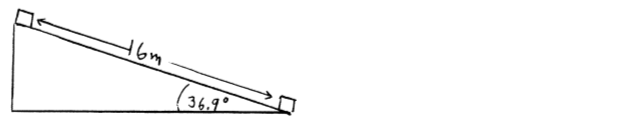# Problem: A block of mass 10.0 kg slides 16.0 m down a 36.9° incline, from point A at the top of the incline to point B at the bottom. As the block moves from point A to point B, the surface of the incline exerts a constant friction force that has magnitude 42.0 N.If the block has an initial speed of 8.0 m/s at point A, what is the speed of the block when it reaches point B?

###### Problem Details

A block of mass 10.0 kg slides 16.0 m down a 36.9° incline, from point A at the top of the incline to point B at the bottom. As the block moves from point A to point B, the surface of the incline exerts a constant friction force that has magnitude 42.0 N.

If the block has an initial speed of 8.0 m/s at point A, what is the speed of the block when it reaches point B?